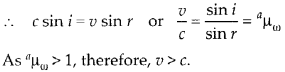Enlightened

# Question 12: NCERT Solutions for 12th Class Physics: Chapter 10-Wave Optics

• 0

Question 12: NCERT Solutions for 12th Class Physics: Chapter 10-Wave Optics

Explain how corpuscular theory predicts that the speed of light in a medium, say, water, is greater than the speed of light in vacuum. Is the prediction confirmed by experimental determination of the speed of light in water? If not, which alternative picture of light is consistent with experiment?

Share

1. Solution:
In Newton’s corpuscular (particle) picture of refraction, particles of light incident from a rarer to a denser medium experience a force of attraction normal to the surface. This results in an increase in the normal component of velocity but the component along the surface remains unchanged.Considering a ray of light going from a rarer medium (air) to a denser medium (water). Let c = speed of light in vacuum (or air), v = speed of light in water, i = angle of incidence, and r = angle of refraction Then according to Newton’s corpuscular theory,

Component of velocity c along surface of separation = Component of velocity v along the surface of separationSo, according to Newton’s corpuscular theory the speed of light in medium is larger than speed of light in air. υ > c but in fact the experimental observation shows that speed of light is smaller in denser medium as compared to rare medium υ < c.

Check the complete chapter with solutions.

NCERT Solutions for 12th Class Physics: Chapter 10-Wave Optics

• 0